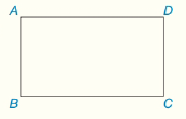Chapter 4.CT, Problem 11CT### Elementary Geometry for College St...

6th Edition
Daniel C. Alexander + 1 other
ISBN: 9781285195698

#### Solutions

Chapter
Section### Elementary Geometry for College St...

6th Edition
Daniel C. Alexander + 1 other
ISBN: 9781285195698
Textbook Problem
1 views

# In rectangle ABCD, AD=12 and DC=5. Find the length of diagonal A C ¯ (not shown).                                     _To determine

To Find:

Length of AC.

Explanation

Given:

ABCD is a rectangle and AD = 12 and DC = 5.

Calculation:

All interior angles in a rectangle are right angle.

D is a right angle and hence ΔACD is a right triangle with hypotenuse AC.

By Pythagorean Theorem in ΔACD,

AC2

### Still sussing out bartleby?

Check out a sample textbook solution.

See a sample solution

#### The Solution to Your Study Problems

Bartleby provides explanations to thousands of textbook problems written by our experts, many with advanced degrees!

Get Started

#### Find more solutions based on key concepts